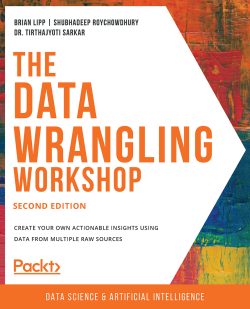# The Data Wrangling Workshop - Second Edition

By Brian Lipp , Shubhadeep Roychowdhury , Dr. Tirthajyoti Sarkar
• \$5/mo for 5 months Subscribe Access now
• \$34.99 Print + eBook
• \$24.99 eBook

• Constantly updated with 100+ new titles each month
• Breadth and depth in over 1,000+ technologies

While a huge amount of data is readily available to us, it is not useful in its raw form. For data to be meaningful, it must be curated and refined.

If you’re a beginner, then The Data Wrangling Workshop will help to break down the process for you. You’ll start with the basics and build your knowledge, progressing from the core aspects behind data wrangling, to using the most popular tools and techniques.

This book starts by showing you how to work with data structures using Python. Through examples and activities, you’ll understand why you should stay away from traditional methods of data cleaning used in other languages and take advantage of the specialized pre-built routines in Python. Later, you’ll learn how to use the same Python backend to extract and transform data from an array of sources, including the internet, large database vaults, and Excel financial tables. To help you prepare for more challenging scenarios, the book teaches you how to handle missing or incorrect data, and reformat it based on the requirements from your downstream analytics tool.

By the end of this book, you will have developed a solid understanding of how to perform data wrangling with Python, and learned several techniques and best practices to extract, clean, transform, and format your data efficiently, from a diverse array of sources.

Publication date:
July 2020
Publisher
Packt
Pages
576
ISBN
9781839215001

# 1. Introduction to Data Wrangling with Python

Overview

This chapter will help you understand the importance of data wrangling in data science. You will gain practical knowledge of how to manipulate the data structures that are available in Python by comparing the different implementations of the built-in Python data structures. Overall, this chapter describes the importance of data wrangling, identifies the important tasks to be performed in data wrangling, and introduces basic Python data structures. By the end of this chapter, you will be adept at working with lists, sets, and dictionaries, which are the key building blocks of data structures in Python.

# Introduction

Since data science and analytics have become key parts of our lives, the role of a data scientist has become even more important. Finding the source of data is an essential part of data science; however, it is the science part that makes you – the practitioner – truly valuable.

To practice high-quality science with data, you need to make sure it is properly sourced, cleaned, formatted, and pre-processed. This book will teach you the most essential basics of this invaluable component of the data science pipeline: data wrangling. In short, data wrangling is the process that ensures that the data is being presented in a way that is clean, accurate, formatted, and ready to be used for data analysis.

A prominent example of data wrangling with a large amount of data is the analysis conducted at the Supercomputer Center of the University of California San Diego (UCSD) every year. Wildfires are very common in California and are caused mainly by the dry weather and extreme heat, especially during the summers. Data scientists at the UCSD Supercomputer Center run an analysis every year and gather data to predict the nature and spread direction of wildfires in California. The data comes from diverse sources, such as weather stations, sensors in the forest, fire stations, satellite imagery, and Twitter feeds. However, this data might be incomplete or missing.

After collecting the data from various sources, if it is not cleaned and formatted using ways including scaling numbers and removing unwanted characters in strings, it could result in erroneous data. In cases where we might get a flawed analysis, we might need to reformat the data from JavaScript Object Notation (JSON) into Comma Separated Value (CSV); we may also need the numbers to be normalized, that is, centered and scaled with relation to themselves. Processing data in such a way might be required when we feed data to certain machine learning models.

This is an example of how data wrangling and data science can prove to be helpful and relevant. This chapter will discuss the fundamentals of data wrangling. Let's get started.

# Importance of Data Wrangling

A common mantra of the modern age is Data is the New Oil, meaning data is now a resource that's more valuable than oil. But just as crude oil does not come out of the rig as gasoline and must be processed to get gasoline and other products, data must be curated, massaged, or cleaned and refined to be used in data science and products based on data science. This is known as wrangling. Most data scientists spend the majority of their time data wrangling.

Data wrangling is generally done at the very first stage of a data science/analytics pipeline. After the data scientists have identified any useful data sources for solving the business problem at hand (for instance, in-house database storage, the internet, or streaming sensor data such as an underwater seismic sensor), they then proceed to extract, clean, and format the necessary data from those sources.

Generally, the task of data wrangling involves the following steps:

1. Scraping raw data from multiple sources (including web and database tables)
2. Imputing (replacing missing data using various techniques), formatting, and transforming – basically making it ready to be used in the modeling process (such as advanced machine learning)
4. Detecting outliers
5. Performing quick visualizations (plotting) and basic statistical analysis to judge the quality of formatted data

The following is an illustrative representation of the positioning and the essential functional role of data wrangling in a typical data science pipeline: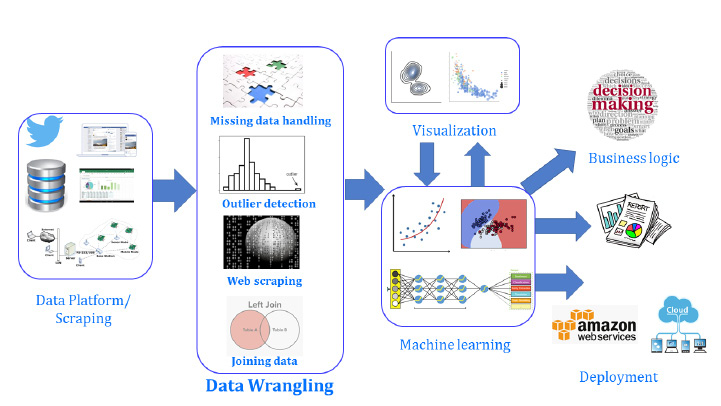Figure 1.1: Process of data wrangling

The process of data wrangling includes finding the appropriate data that's necessary for the analysis. Often, analysis is exploratory, so there is not enough scope. You often need to do data wrangling for this type of analysis to be able to understand your data better. This could lead to more analysis or machine learning.

This data can be from one or multiple sources, such as tweets, bank transaction statements in a relational database, sensor data, and so on. This data needs to be cleaned. If there is missing data, we will either delete or substitute it, with the help of several techniques. If there are outliers, we need to detect them and then handle them appropriately. If the data is from multiple sources, we will have to combine it using Structured Query Language (SQL) operations like JOIN.

In an extremely rare situation, data wrangling may not be needed. For example, if the data that's necessary for a machine learning task is already stored in an acceptable format in an in-house database, then a simple SQL query may be enough to extract the data into a table, ready to be passed on to the modeling stage.

# Python for Data Wrangling

There is always a debate regarding whether to perform the wrangling process using an enterprise tool or a programming language and its associated frameworks. There are many commercial, enterprise-level tools for data formatting and preprocessing that do not involve much coding on the user's part. Some of these examples include the following:

• General-purpose data analysis platforms, such as Microsoft Excel (with add-ins)
• Statistical discovery package, such as JMP (from SAS)
• Modeling platforms, such as RapidMiner
• Analytics platforms from niche players that focus on data wrangling, such as Trifacta, Paxata, and Alteryx

However, programming languages such as Python and R provide more flexibility, control, and power compared to these off-the-shelf tools. This also explains their tremendous popularity in the data science domain: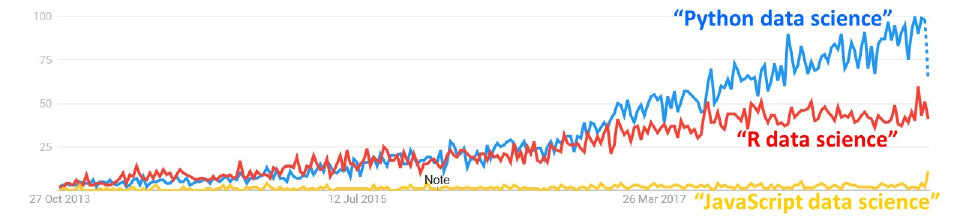Figure 1.2: Google trends worldwide over the last 5 years

Furthermore, as the volume, velocity, and variety (the three Vs of big data) of data undergo rapid changes, it is always a good idea to develop and nurture a significant amount of in-house expertise in data wrangling using fundamental programming frameworks so that an organization is not beholden to the whims and fancies of any particular enterprise platform for as basic a task as data wrangling.

A few of the obvious advantages of using an open source, free programming paradigm for data wrangling are as follows:

• A general-purpose open-source paradigm puts no restrictions on any of the methods you can develop for the specific problem at hand.
• There's a great ecosystem of fast, optimized, open-source libraries, focused on data analytics.
• There's also growing support for connecting Python to every conceivable data source type.
• There's an easy interface to basic statistical testing and quick visualization libraries to check data quality.
• And there's a seamless interface of the data wrangling output with advanced machine learning models.

Python is the most popular language for machine learning and artificial intelligence these days. Let's take a look at a few data structures in Python.

# Lists, Sets, Strings, Tuples, and Dictionaries

Now that we have touched upon a few advantages of using Python, we will start by exploring various basic data structures in Python. We will also learn about a few techniques we can use to handle these data structures. This is invaluable for a data practitioner.

## Lists

Lists are fundamental Python data structures that have continuous memory locations and can host different data types (such as strings, numbers, floats, and doubles) and can be accessed by the index.

We will start with a list and list comprehension. A list comprehension is a syntactic sugar (or shorthand) for a `for` loop, which iterates over a list. We will generate a list of numbers, and then examine which ones among them are even. We will sort, reverse, and check for duplicates. We will also see the different ways we can access the list elements, iterating over them and checking the membership of an element.

The following is an example of a simple list:

`list_example = [51, 27, 34, 46, 90, 45, -19]`

The following is also an example of a list:

`list_example2 = [15, "Yellow car", True, 9.456, [12, "Hello"]]`

As you can see, a list can contain any number of the allowed data types, such as `int`, `float`, `string`, and `boolean`, and a list can also be a mix of different data types (including nested lists).

If you are coming from a strongly typed language, such as C, C++, or Java, then this will probably be strange as you are not allowed to mix different kinds of data types in a single array in those languages. Lists in Python are loosely typed, that is, they are not restricted to a single type. Lists are somewhat like arrays in the sense that they are both based on continuous memory locations and can be accessed using indexes. But the power of Python lists comes from the fact that they can host different data types and you are allowed to manipulate the data.

In Python, there is a concept of creating a slice of a list. Here is the syntax:

`my_list [ inclusive start index : exclusive end index ]`

Known as list slicing, this returns a smaller list from the original list by extracting only a part of it. To slice a list, we need two integers. The first integer will denote the start of the slice and the second integer will denote the end. Notice that slicing does not include the third index or the end element. A slice is a chunk of the list tuple or string. The range is from `0` to `1` minus the total length. The first number given represents the first position to include in the slice. The second number is used to indicate which place you want to stop at, but not include. A slice can have an index of `–1` to indicate the last element.

The indices will be automatically assigned, as follows: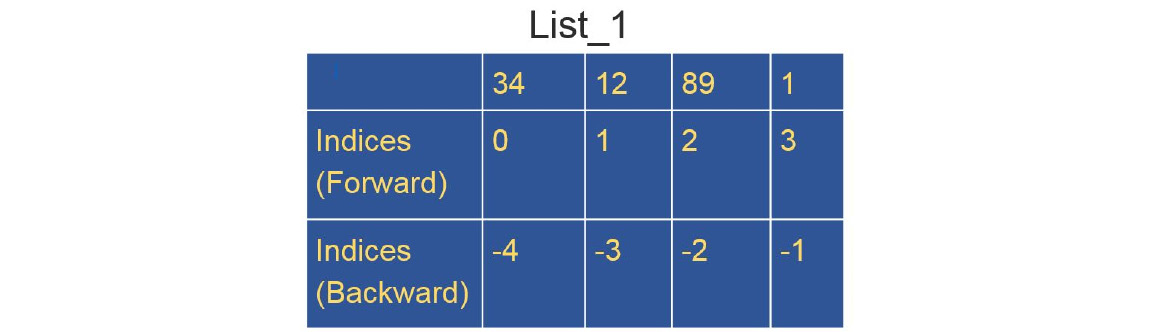Figure 1.3: List showing the forward and backward indices

Note

Be careful, though, as the very power of lists, and the fact that you can mix different data types in a single list, can actually create subtle bugs that can be very difficult to track.

# List Functions

In this section, we will discuss a few basic functions for handling lists.

You can access list elements using the following code:

```list_example = [51, 27, 34, 46, 90, 45, -19]
list_example```

The output is as follows:

`51`

To find out the length of a list, we simply use the `len` function. The `len` function in Python returns the length of the specified list:

`len(list_example)`

The output is as follows:

`7`

We can append new elements in the list. `append` is a built-in method in Python for the list data type:

```list_example.append(11)
list_example```

The output is as follows:

`[51, 27, 34, 46, 90, 45, -19, 11]`

Let's go through some exercises to practice these functions.

## Exercise 1.01: Accessing the List Members

In this exercise, we will be reading a list of social security numbers from the `ssn.csv` file and then observing the different ways of accessing the elements from the list using the forward and backward indices. We will be using our first Python function, `len`, which will return the length of the specified list. We will be starting with the basic building blocks for future exercises. Let's go through the following steps:

Note

The `ssn.csv` file can be found here: https://packt.live/2AydLCx.

1. Open a new Jupyter Notebook and define a list called `ssn`. Read from the `ssn.csv` file using the `read_csv` command and print the list elements:
```import pandas as pd
print(ssn)```

Note

The highlighted path in the code must be changed based on where you have saved the `ssn.csv` file on your system.

The output is as follows:

```['218-68-9955',
'165-73-3124',
'432-47-4043',
'563-93-1393',
'153-93-3401',
'670-09-7369',
'123-05-9652',
'812-13-2476',
'726-13-1007',
'825-05-4836']```
2. Access the first element of `ssn` using its forward index:
`ssn`

The output is as follows:

`'218-68-9955'`
3. Access the fourth element of `ssn` using its forward index:
`ssn`

The output is as follows:

`'563-93-1393'`
4. Access the last element of `ssn` using the `len` function:
`ssn[len(ssn) - 1]`

The output is as follows:

`'825-05-4836'`
5. Access the last element of `ssn` using its backward index:
`ssn[-1] `

The output is as follows:

`'825-05-4836'`
6. Access the first three elements of `ssn` using forward indices:
`ssn[1:3]`

The output is as follows:

`['165-73-3124', '432-47-4043']`
7. Access the last two elements of `ssn` by slicing:
`ssn[-2:]`

The output is as follows:

`['726-13-1007', '825-05-4836']`
8. Access the first two elements using backward indices:
`ssn[:-2]`

The output is as follows:Figure 1.4: Output after using backward indices

When we leave one side of the colon (`:`) blank, we are basically telling Python either to go until the end or start from the beginning of the list. It will automatically apply the rule of list slices that we just learned.

9. Reverse the elements in the list:
`ssn[-1::-1]`

The output is as follows:Figure 1.5: Output after elements are reversed

Note

To access the source code for this specific section, please refer to https://packt.live/3fzTvzk.

You can also run this example online at https://packt.live/2YF3zA0.

In this exercise, we learned how to access the list members with forward and backward indices. We'll create a list in the next exercise.

## Exercise 1.02: Generating and Iterating through a List

In this exercise, we are going to examine various ways of generating a list and a nested list using the same file containing the list of social security numbers (`ssn.csv`) that we used in the previous exercise.

Note

The `ssn.csv` file can be found here: https://packt.live/2AydLCx.

We are going to use the `append` method to add new elements to the list and a `while` loop to iterate through the list. To do so, let's go through the following steps:

1. Open a new Jupyter Notebook and import the necessary Python libraries. Read from the `ssn.csv` file:
```import pandas as pd

Note

The highlighted path in the code must be changed based on where you have saved the `ssn.csv` file on your system.

2. Create a list using the `append` method. The `append` method from the Python library will allow you to add items to the list:
```ssn_2 = []
for x in ssn:
ssn_2.append(x)
ssn_2```

The output will be as follows:Figure 1.6: Output after creating a list using the append method

Here, we started by declaring an empty list called `ssn_2`, and then we used a `for` loop to append values to it after reading from the `ssn.csv` file.

3. Generate a list using the following command:
```ssn_3 = ["soc: " + x for x in ssn_2]
ssn_3```

The output is as follows:Figure 1.7: Output of a generated list

This is list comprehension, which is a very powerful tool that we need to master. The power of list comprehension comes from the fact that we can use conditionals such as `for..in` inside the comprehension itself. This will be discussed in detail in Chapter 2, Advanced Operations on Built-in Data Structures.

4. Use a `while` loop to iterate over the list:

Note

Conditionals work the same way as in any other strongly typed language, such as C, C++, or Java.

```i = 0
while i < len(ssn_3):
print(ssn_3[i])
i += 1```

The output is as follows:

```soc: 218-68-9955
soc: 165-73-3124
soc: 432-47-4043
soc: 563-93-1393
soc: 153-93-3401
soc: 670-09-7369
soc: 123-05-9652
soc: 812-13-2476
soc: 726-13-1007
soc: 825-05-4836```
5. Search all the social security numbers with the number `5` in them:
```numbers = [x for x in ssn_3 if "5" in x]
numbers```

The output will be as follows:Figure 1.8: SSNs with the number 5 in them

Let's explore a few more list operations. We are going to use the `+` operator to add the contents of two lists and use the `extend` keyword to replace the contents of the existing list with another list.

6. Generate a list by adding the two lists. Here, we will just use the `+` operator:
```ssn_4 = ["102-90-0314" , "247-17-2338" , "318-22-2760"]
ssn_5 = ssn_4 + ssn
ssn_5```

The output is as follows: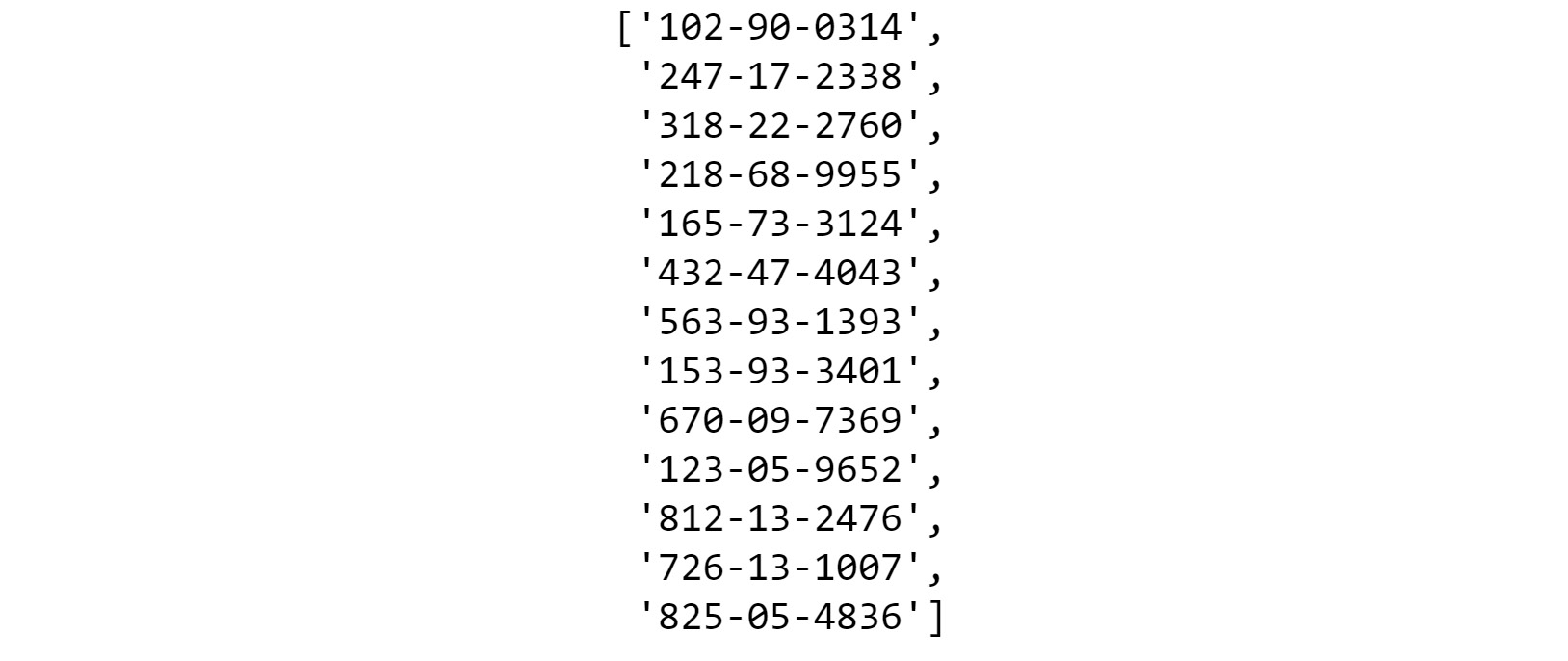Figure 1.9: Generated list by adding two lists

7. Extend a string using the `extend` keyword:
```ssn_2.extend(ssn_4)
ssn_2```

The output is as follows:Figure 1.10: The extend string operation

Note

The `extend` operation changes the original list (`ssn_2`) and appends all the elements of `ssn_4` to it. So, be careful while using it.

8. Now, let's loop over the first list and create a nested list inside that loop that goes over the second list:
```for x in ssn_2:
for y in ssn_5:
print(str(x) + ' , ' + str(y))```

The output (partially shown) is as follows: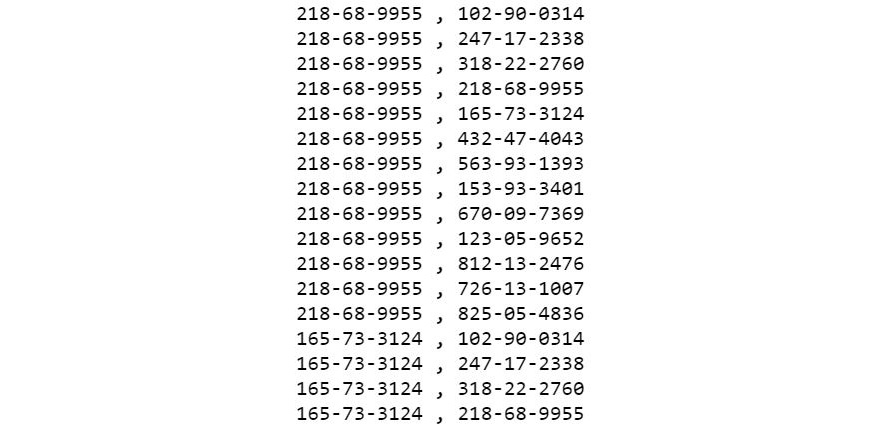Figure 1.11: Partial output of ssn

Note

To access the source code for this specific section, please refer to https://packt.live/2Y6vObR.

You can also run this example online at https://packt.live/2YLJybf.

In this exercise, we used the built-in methods of Python to manipulate lists. In the next exercise, we'll check whether the elements or members in a dataset are present as per our expectations.

## Exercise 1.03: Iterating over a List and Checking Membership

This exercise will demonstrate how we can iterate over a list and verify that the values are as expected. This is a manual test that can often be done while dealing with a reasonably sized dataset for business case scenarios. Let's go through the following steps to check the membership of values and whether they exist in the `.csv` file:

Note

The `car_models.csv` file can be found at https://packt.live/3d8DUVy.

1. Import the necessary Python libraries and read from the `car_models.csv` file:
```import pandas as pd
car_models```

Note

The highlighted path in the code must be changed based on where you have saved the `car_models.csv` file on your system.

The output is as follows:

```['Escalade ',
‹ X5 M›,
‹D150›,
‹Camaro›,
‹F350›,
‹Aurora›,
‹S8›,
‹E350›,
‹Tiburon›,
‹F-Series Super Duty ‹]```
2. Iterate over a list:
```list_1 = [x for x in car_models]
for i in range(0, len(list_1)):
print(list_1[i])```

The output is as follows:

```Escalade
X5 M
D150
Camaro
F350
Aurora
S8
E350
Tiburon
F-Series Super Duty```

However, this is not very Pythonic. Being Pythonic means to follow and conform to a set of best practices and conventions that have been created over the years by thousands of capable developers. In this case, this means we could use the `in` keyword in the `for..in` conditional because Python does not have index initialization, bounds checking, or index incrementing, unlike traditional languages. Python uses syntactic sugar to make iterating through lists easy and readable. In other languages, you might have to create a variable (index initialization) as you loop over the list check that variable (bounds checking) since it will be incremented in the loop (index incrementing).

3. Write the following code to see the Pythonic way of iterating over a list:
```for i in list_1:
print(i)```

The output is as follows:

```Escalade
X5 M
D150
Camaro
F350
Aurora
S8
E350
Tiburon
F-Series Super Duty```

Notice that in the second method, we do not need a counter anymore to access the list index; instead, Python's `in` operator gives us the element at the `i`th position directly.

4. Check whether the strings `D150` and `Mustang` are in the list using the `in` operator:
`"D150" in list_1`

The output is `True`.

`"Mustang" in list_1`

The output is `False`.

Note

To access the source code for this specific section, please refer to https://packt.live/30TpGp5.

You can also run this example online at https://packt.live/2Y8z06L.

In this exercise, we've seen how to iterate over a list and verified the membership of each element. This is an important skill. Often, when working with large applications, manually checking a list could be useful. If at any time you are unsure of a list, you can easily verify what values are present. Now, we will see how we can perform a sort operation on a list.

## Exercise 1.04: Sorting a List

In this exercise, we will sort a list of numbers, first by using the `sort` method and then by using the `reverse` method. To do so, let's go through the following steps:

Note

The `ssn.csv` file can be found here: https://packt.live/2AydLCx.

1. Open a new Jupyter Notebook and import the necessary Python libraries:
```import pandas as pd

Note

The highlighted path in the code must be changed based on where you have saved the `ssn.csv` file on your system.

2. Use the `sort` method with `reverse=True`:
```list_1 = [*range(0, 21, 1)]
list_1.sort(reverse=True)
list_1```

The output is as follows:

```[20, 19, 18, 17, 16, 15, 14, 13, 12, 11, 10, 9, 8, 7,
6, 5, 4, 3, 2, 1, 0]```
3. Use the `reverse` method directly to achieve this result:
```list_1.reverse()
list_1```

The output is as follows:

```[0, 1, 2, 3, 4, 5, 6, 7, 8, 9, 10, 11, 12, 13, 14, 15, 16,
17, 18, 19, 20]```

Note

To access the source code for this specific section, please refer to https://packt.live/2Y7HlYe.

You can also run this example online at https://packt.live/2YGNvOd.

The difference between the `sort` method and the `reverse` method is that we can use `sort` with customized sorting, whereas we can only use `reverse` to reverse a list. Also, both methods work in-place, so be aware of this while using them. Now, let's create a list with random numbers. Random numbers can be very useful in a variety of situations and preprocessing data is a common process in machine learning.

## Exercise 1.05: Generating a Random List

In this exercise, we will be generating a `list` with random numbers using the `random` library in Python and performing mathematical operations on them. To do so, let's go through the following steps:

1. Import the `random` library:
`import random`
2. Use the `randint` method to generate some random integers and add them to a list:
`list_1 = [random.randint(0, 30) for x in range (0, 100)]`
3. Let's print the list. Note that there will be duplicate values in `list_1`:
`list_1`

The sample output (partially shown) is as follows:Figure 1.12: List of random numbers

Note

The output will vary with every run since we are generating random numbers.

4. Let's find the square of each element:
```list_2 = [x**2 for x in list_1]
list_2```

The output is as follows:Figure 1.13: List of random numbers

5. Now let's find the log of the `1` elements of `list_2`:
```import math
list_2 = [math.log(x+1,10) for x in list_2]
list_2```

The output (partially shown) is as follows: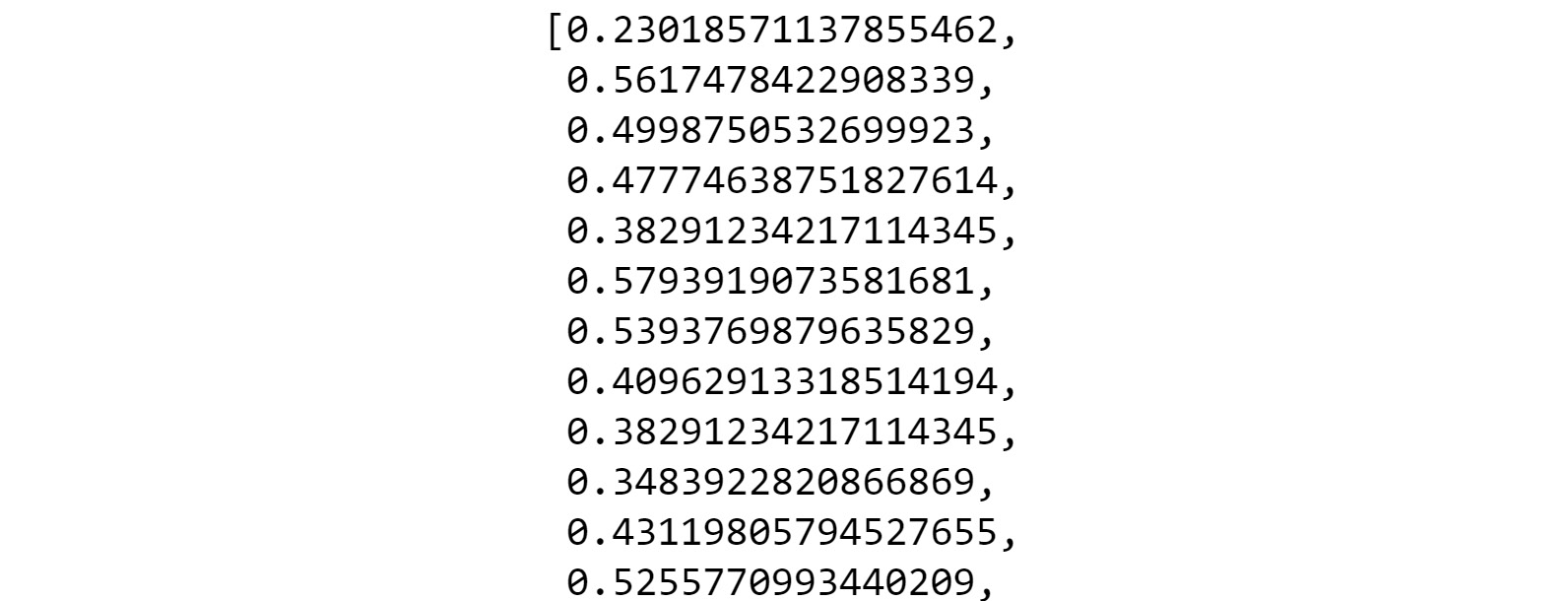Figure 1.14: Partial output for list_2

Note

The output is susceptible to change since we are generating random numbers.

Note

To access the source code for this specific section, please refer to https://packt.live/37BerTD.

You can also run this example online at https://packt.live/3hHwlsH.

In this exercise, we worked on random variables, lists comprehension, and preprocessing data. Let's put what we have learned so far together and go through an activity to practice how to handle lists.

## Activity 1.01: Handling Lists

In this activity, you will generate a list of random numbers and then generate another list from the first one, which only contains numbers that are divisible by three. Repeat the experiment 10 times; you'll see that the output varies each time, given that a different set of random numbers will be generated each time. Then, you will calculate the average difference between the lengths of the two lists.

These are the steps for completing this activity:

1. Create a `list` of `100` random numbers.
2. Create a new `list` from this random `list`, with numbers that are divisible by `3`.
3. Calculate the length of these two lists and store the difference in a new variable.
4. Using a loop, perform steps 1, 2, and 3, and find the difference variable 10 times.
5. Find the arithmetic mean of these `10` difference values.

The output (will vary with each run) should look similar to this:

`66.3`

Note

The solution for this activity can be found via this link.

## Sets

A set, mathematically speaking, is just a collection of well-defined distinct objects. Python gives us a straightforward way to deal with them using its set data type.

## Introduction to Sets

With the last list that we generated in the previous section; we are going to revisit the problem of getting rid of duplicates from it. We can achieve that with the following line of code:

`list_12 = list(set(list_1))`

If we print this, we will see that it only contains unique numbers. We used the set data type to turn the first list into a set, thus getting rid of all duplicate elements, and then used the list function to turn it into a list from a set once more:

`list_12`

The output will be as follows:Figure 1.15: Section of output for list_12

In the next section, we will discuss the union and intersection of sets.

## Union and Intersection of Sets

In mathematical terms, a list of unique objects is a set. There are many ways of combining sets in the same mathematical term. One such way is the use of a union.

This is what a union between two sets looks like:Figure 1.16: Venn diagram showing the union of two sets

This simply means taking everything from both sets but only taking the common elements once.

We can implement this concept by using the following code:

```set1 = {"Apple", "Orange", "Banana"}
set2 = {"Pear", "Peach", "Mango", "Banana"}```

To find the union of the two sets, the following code should be used:

`set1 | set2`

The output would be as follows:

`{'Apple', 'Banana', 'Mango', 'Orange', 'Peach', 'Pear'}`

Notice that the common element, `Banana`, appears only once in the resulting set. The common elements of two sets can be identified by obtaining the intersection of the two sets, as follows:Figure 1.17: Venn diagram showing the intersection of two sets

We get the intersection of two sets in Python as follows:

`set1 & set2`

This will give us a set with only one element. The output is as follows:

`{'Banana'}`

Note

You can also calculate the difference between sets (also known as complements). To find out more, refer to this link: https://docs.python.org/3/tutorial/datastructures.html#sets.

In this section, we went through sets and how we can do basic set functionality. Sets are used throughout database programming and design, and they are very useful for data wrangling.

## Creating Null Sets

In mathematical terms, a set that has nothing inside it is called a null set or an empty set.

You can create a null set by creating a set containing no elements. You can do this by using the following code:

```null_set_1 = set({})
null_set_1```

The output is as follows:

`set()`

However, to create a dictionary with null values, use the following command:

```null_set_2 = {}
null_set_2```

The output is as follows:

`{}`

## Dictionary

A dictionary is like a list, which means it is a collection of several elements. However, with the dictionary, it is a collection of key-value pairs, where the key can be anything that can fit into memory. Generally, we use numbers or strings as keys.

To create a dictionary, use the following code:

```dict_1 = {"key1": "value1", "key2": "value2"}
dict_1```

The output is as follows:

`{'key1': 'value1', 'key2': 'value2'}`

This is also a valid dictionary:

```dict_2 = {"key1": 1, "key2": ["list_element1", 34], \
"key3": "value3","key4": {"subkey1": "v1"}, \
"key5": 4.5}
dict_2```

Note

The code snippet shown here uses a backslash ( `\` ) to split the logic across multiple lines. When the code is executed, Python will ignore the backslash, and treat the code on the next line as a direct continuation of the current line.

The output is as follows:

```{'key1': 1,
'key2': ['list_element1', 34],
'key3': 'value3',
'key4': {'subkey1': 'v1'},
'key5': 4.5}```

The keys must be unique in a dictionary.

## Exercise 1.06: Accessing and Setting Values in a Dictionary

In this exercise, we are going to access the elements and set values in a dictionary. When working with dictionaries, it's important to be able to iterate through each key-value pair, which will allow you to process the data as needed. To do so, let's go through the following steps:

1. To access a value in the dictionary, you must provide the key. Keep in mind there is no given order for any pair in the dictionary:
```stocks = \
{"Solar Capital Ltd.":"\$920.44M", \
"Zoe's Kitchen, Inc.":"\$262.32M",\
"Toyota Motor Corp Ltd Ord":"\$156.02B",\
"Nuveen Virginia Quality Municipal Income Fund":"\$238.33M",\
"Kinross Gold Corporation":"\$5.1B",\
"Vulcan Materials Company":"\$17.1B",\
"Hi-Crush Partners LP":"\$955.69M",\
"Lennox International, Inc.":"\$8.05B",\
"WMIH Corp.":"\$247.66M",\
"Comerica Incorporated":"n/a"}```
2. Print a particular element from the `stocks` list:
`stocks["WMIH Corp."]`

This will return the value associated with it, as follows:

`'\$247.66M'`
3. Set a value using the same method we use to access a value:
`stocks["WMIH Corp."] = "\$300M"`
4. Define a blank dictionary and then use the key notation to assign values to it:
```dict_3 = {} # Not a null set. It is a dict
dict_3["key1"] = "Value1"
dict_3```

Note

The `#` symbol in the code snippet above denotes a code comment. Comments are added into code to help explain specific bits of logic.

The output is as follows:

`{'key1': 'Value1'}`

Note

To access the source code for this specific section, please refer to https://packt.live/2AGFaly.

You can also run this example online at https://packt.live/3d8fyeJ.

As we can see, the manipulation techniques of a dictionary are pretty simple. Now, just like a list, iterating through a dictionary is very important in order to process the data.

## Exercise 1.07: Iterating over a Dictionary

In this exercise, we are going to iterate over a dictionary and print the values and keys. To do so, let's go through the following steps:

1. Open a new Jupyter Notebook and define a dictionary with the key provided along with it. Keep in mind there is no given order for any pair in the dictionary:
```stocks = \
{"Solar Capital Ltd.":"\$920.44M",\
"Zoe's Kitchen, Inc.":"\$262.32M",\
"Toyota Motor Corp Ltd Ord":"\$156.02B",\
"Nuveen Virginia Quality Municipal Income Fund":"\$238.33M",\
"Kinross Gold Corporation":"\$5.1B",\
"Vulcan Materials Company":"\$17.1B",\
"Hi-Crush Partners LP":"\$955.69M",\
"Lennox International, Inc.":"\$8.05B",\
"WMIH Corp.":"\$247.66M",\
"Comerica Incorporated":"n/a"}```
2. Remove the `\$` character from the `stocks` dictionary:
```for key,val in stocks.items():
stocks[key] = val.replace('\$', '')
stocks```

The output should be as follows:

```{'Solar Capital Ltd.': '920.44M',
"Zoe's Kitchen, Inc.": '262.32M',
'Toyota Motor Corp Ltd Ord': '156.02B',
'Nuveen Virginia Quality Municipal Income Fund': '238.33M',
'Kinross Gold Corporation': '5.1B',
'Vulcan Materials Company': '17.1B',
'Hi-Crush Partners LP': '955.69M',
'Lennox International, Inc.': '8.05B',
'WMIH Corp.': '300M',
'Comerica Incorporated': 'n/a'}```
3. Iterate over the `stocks` dictionary again and split the value into a list with price (`val`) and multiplier (`mult`) as separate elements where a single value is assigned to each key:
```for key,val in stocks.items():
mult = val[-1]
stocks[key] = [val[:-1],mult]
stocks```

The output is as follows:

```{'Solar Capital Ltd.': ['920.44', 'M'],
«Zoe›s Kitchen, Inc.»: [‹262.32›, ‹M›],
‹Toyota Motor Corp Ltd Ord›: [‹156.02›, ‹B›],
‹Nuveen Virginia Quality Municipal Income Fund›: [‹238.33›, ‹M›],
‹Kinross Gold Corporation›: [‹5.1›, ‹B›],
‹Vulcan Materials Company›: [‹17.1›, ‹B›],
‹Hi-Crush Partners LP›: [‹955.69›, ‹M›],
‹Lennox International, Inc.›: [‹8.05›, ‹B›],
‹WMIH Corp.›: [‹300›, ‹M›],
‹Comerica Incorporated›: [‹n/›, ‹a›]}```

Notice the difference between how we did the iteration on the list and how we are doing it here. A dictionary always contains a key-value pair, and we always need to access the value of any element in a dictionary with its key. In a dictionary, all the keys are unique.

Note

To access the source code for this specific section, please refer to https://packt.live/3db0xZF.

You can also run this example online at https://packt.live/2zDFHnU.

In the next exercise, we will revisit the problem that we encountered with the list earlier in this chapter to create a list with unique values. We will look at another workaround to fix this problem.

## Exercise 1.08: Revisiting the Unique Valued List Problem

In this exercise, we will use the unique nature of a dictionary, and we will drop the duplicate values from a list. First, we will create a random list with duplicate values. Then, we'll use the `fromkeys` and `keys` methods of a dictionary to create a unique valued list. To do so, let's go through the following steps:

1. First, generate a random list with duplicate values:
```import random
list_1 = [random.randint(0, 30) for x in range (0, 100)]```
2. Create a unique valued list from `list_1`:
`list(dict.fromkeys(list_1).keys())`

The sample output is as follows: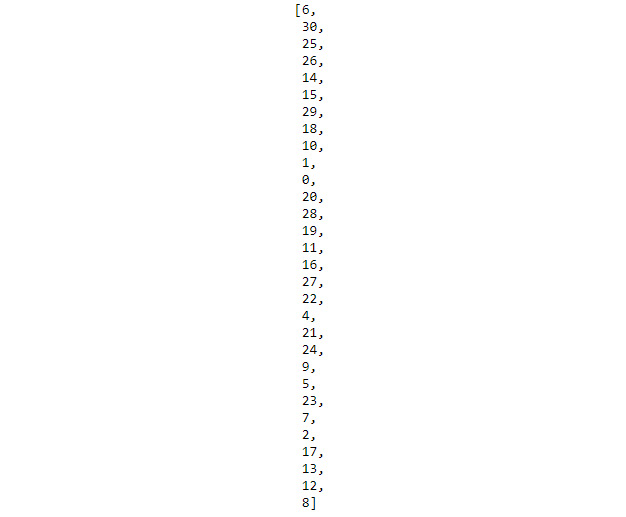Figure 1.18: Output showing the unique valued list

Note

The output is susceptible to change since we are generating random numbers.

Here, we have used two useful methods of the `dict` data type in Python, `fromkeys` and `keys`. `fromkeys` is a built-in function in which a new dictionary is created from the given sequence of elements with values given by the user, while the `keys` method gives us the keys of a dictionary.

Note

To access the source code for this specific section, please refer to https://packt.live/2URp6EA.

You can also run this example online at https://packt.live/2UTCFmO.

## Exercise 1.09: Deleting a Value from Dict

In this exercise, we are going to delete a value from `dict` using the `del` method. Perform the following steps:

1. Create `list_1` with five elements:
```dict_1 = {"key1": 1, "key2": ["list_element1", 34], \
"key3": "value3","key4": {"subkey1": "v1"}, \
"key5": 4.5}
dict_1```

The output is as follows:

```{'key1': 1,
'key2': ['list_element1', 34],
'key3': 'value3',
'key4': {'subkey1': 'v1'},
'key5': 4.5}```
2. We will use the `del` function and specify the element we want to delete:
```del dict_1["key2"]
dict_1```

The output is as follows:

`{'key1': 1, 'key3': 'value3', 'key4': {'subkey1': 'v1'}, 'key5': 4.5}`
3. Let's delete `key3` and `key4`:
```del dict_1["key3"]
del dict_1["key4"]```
4. Now, let's print the dictionary to see its content:
`dict_1`

The output should be as follows:

`{'key1': 1, 'key5': 4.5}`

Note

To access the source code for this specific section, please refer to https://packt.live/2Nb3oqF.

You can also run this example online at https://packt.live/30Os7ct.

In this exercise, we learned how to delete elements from a dictionary. This is a very useful functionality of dictionaries, and you will find that it's used heavily when writing Python applications.

Note

The `del` operator can be used to delete a specific index from a list as well.

In our final exercise on `dict`, we will go over a less commonly used list comprehension called dictionary comprehension. We will also examine two other ways to create a `dict`, which can be very useful for processing dictionaries in one line. There could be cases where this could be used as a range of key-value pairs of name and age or credit card number and credit card owner. A dictionary comprehension works exactly the same way as list comprehension, but we need to specify both the key and the value.

## Exercise 1.10: Dictionary Comprehension

In this exercise, we will generate a dictionary using the following steps:

1. Generate a `dict` that has `0` to `9` as the keys and the square of the key as the values:
```list_1 = [x for x in range(0, 10)]
dict_1 = {x : x**2 for x in list_1}
dict_1```

The output is as follows:

`{0: 0, 1: 1, 2: 4, 3: 9, 4: 16, 5: 25, 6: 36, 7: 49, 8: 64, 9: 81}`

Can you generate a `dict` using `dict` comprehension without using a list? Let's try this now.

2. Generate a `dictionary` using the `dict` function:
```dict_2 = dict([('Tom', 100), ('Dick', 200), ('Harry', 300)])
dict_2```

The output is as follows:

`{'Tom': 100, 'Dick': 200, 'Harry': 300}`
3. You can also a `dictionary` using the `dict` function, as follows:
```dict_3 = dict(Tom=100, Dick=200, Harry=300)
dict_3```

The output is as follows:

`{'Tom': 100, 'Dick': 200, 'Harry': 300}`

Note

To access the source code for this specific section, please refer to https://packt.live/3hz8zPp.

You can also run this example online at https://packt.live/3hA8WJw.

Dictionaries are very flexible and can be used for a variety of tasks. The compact nature of comprehension makes them very popular. The strange-looking pair of values that just looked at (`'Harry', 300`) is called a tuple. This is another important fundamental data type in Python. We will learn about tuples in the next section.

## Tuples

A tuple is another data type in Python. Tuples in Python are similar to lists, with one key difference. A tuple is a variant of a Python list that is immutable. Immutable basically means you can't modify it by adding or removing from the list. It is sequential in nature and similar to lists.

A tuple consists of values separated by commas, as follows:

`tuple_1 = 24, 42, 2.3456, "Hello"`

Notice that, unlike lists, we did not open and close square brackets here.

When referring to a tuple, the length of the tuple is called its cardinality. This comes from database and set theory and is a common way to reference its length.

## Creating a Tuple with Different Cardinalities

This is how we create an empty tuple:

`tuple_1 = ()`

This is how we create a tuple with only one value:

`tuple_1 = "Hello",`

Notice the trailing comma here.

We can nest tuples, similar to lists and dicts, as follows:

```tuple_1 = "hello", "there"
tuple_12 = tuple_1, 45, "Sam"```

One special thing about tuples is the fact that they are an immutable data type. So, once they're created, we cannot change their values. We can just access them, as follows:

```tuple_1 = "Hello", "World!"
tuple_1 = "Universe!"```

The last line of the preceding code will result in a `TypeError` as a tuple does not allow modification.

This makes the use case for tuples a bit different than lists, although they look and behave very similarly in a few ways.

We can access the elements of a tuple in the same manner we can for lists:

```tuple_1 = ("good", "morning!" , "how", "are" "you?")
tuple_1```

The output is as follows:

`'good'`

Let's access another element:

`tuple_1`

The output will be:

`'you?'`

## Unpacking a Tuple

The expression "unpacking a tuple" simply means getting the values contained in the tuple in different variables:

```tuple_1 = "Hello", "World"
hello, world = tuple_1
print(hello)
print(world)```

The output is as follows:

```Hello
World```

Of course, as soon as we do that, we can modify the values contained in those variables.

## Exercise 1.11: Handling Tuples

In this exercise, we will walk through the basic functionalities of tuples. Let's go through the steps one by one:

1. Create a tuple to demonstrate how tuples are immutable. Unpack it to read all the elements, as follows:
```tupleE = "1", "3", "5"
tupleE```

The output is as follows:

`('1', '3', '5')`
2. Try to override a variable from the `tupleE` tuple:
`tupleE = "5"`

This step will result in `TypeError` as the tuple does not allow modification.

```TypeError                                 Traceback (most recent call last)
<ipython-input-58-b4cba6d5ed11> in <module>
----> 1 tupleE = "5"
TypeError: 'tuple' object does not support item assignment```
3. Try to assign a series to the `tupleE` tuple:
`1, 3, 5 = tupleE`

This step will also result in a `SyntaxError`, stating that it can't assign to the literal:

```File "<ipython-input-3-a5283cb38d62>", line 1
1, 3, 5 = tupleE
^
SyntaxError: can't assign to literal```
4. Print variables at `0`th and `1`st positions:
```print(tupleE)
print(tupleE)```

The output is as follows:

```1
3```

Note

To access the source code for this specific section, please refer to https://packt.live/3ebuvOf.

You can also run this example online at https://packt.live/2URh9zo.

We have seen two different types of data so far. One is represented by numbers, while the other is represented by textual data. Now it's time to look into textual data in a bit more detail.

## Strings

In the final section of this chapter, we will learn about strings. Strings in Python are similar to strings in any other programming language.

This is a string:

`string1 = 'Hello World!' `

A string can also be declared in this manner:

`string2 = "Hello World 2!"`

You can use single quotes and double quotes to define a string.

The start and end of a string is defined as:

`str[ inclusive start position:  exclusive end position ]. `

Strings in Python behave similar to lists, apart from one big caveat. Strings are immutable, whereas lists are mutable data structures.

## Exercise 1.12: Accessing Strings

In this exercise, we are going perform mathematical operations to access strings. Let's go through the following steps:

1. Create a string called `str_1`:
```str_1 = "Hello World!"
str_1```

The output is as follows:

`'Hello World!'`

You can access the elements of the string by specifying the location of the element, like we did for lists.

2. Access the first member of the string:
`str_1`

The output is as follows:

`'H'`
3. Access the fifth member of the string:
`str_1`

The output is as follows:

`'o'`
4. Access the last member of the string:
`str_1[len(str_1) - 1]`

The output is as follows:

`'!'`
5. Access the last member of the string, in a different way this time:
`str_1[-1]`

The output is as follows:

`'!'`

Note

To access the source code for this specific section, please refer to https://packt.live/2YHEmF9.

You can also run this example online at https://packt.live/3db191p.

Each of the preceding operations will give you the character at the specific index. The method for accessing the elements of a string is like accessing a list. Let's do a couple of more exercises to manipulate strings.

## Exercise 1.13: String Slices

This exercise will demonstrate how we can slice strings the same way as we did with lists. Although strings are not lists, the functionality will work in the same way.

Let's go through the following steps:

1. Create a string, `str_1`:
```str_1 = "Hello World! I am learning data wrangling"
str_1```

The output is as follows:

`'Hello World! I am learning data wrangling'`
2. Specify the slicing values and slice the string:
`str_1[2:10]`

The output is as follows:

`'llo Worl'`
3. Slice a string by skipping a slice value:
`str_1[-31:]`

The output is as follows:

`'d! I am learning data wrangling'`
4. Use negative numbers to slice the string:
`str_1[-10:-5]`

The output is as follows:

`' wran'`

Note

To access the source code for this specific section, please refer to https://packt.live/2N70Bis.

You can also run this example online at https://packt.live/3d6X9Pu.

As we can see, it is quite simple to manipulate strings with basic operations.

## String Functions

To find out the length of a string, we simply use the `len` function:

```str_1 = "Hello World! I am learning data wrangling"
len(str_1)```

The length of the string is `41`. To convert a string's case, we can use the `lower` and `upper` methods:

```str_1 = "A COMPLETE UPPER CASE STRING"
str_1.lower()```

The output is as follows:

`'a complete upper case string'`

To change the case of the string, use the following code:

`str_1.upper()`

The output is as follows:

`'A COMPLETE UPPER CASE STRING'`

To search for a string within a string, we can use the `find` method:

```str_1 = "A complicated string looks like this"
str_1.find("complicated")
str_1.find("hello")```

The output is `-1`. Can you figure out whether the `find` method is case-sensitive or not? Also, what do you think the `find` method returns when it actually finds the string?

To replace one string with another, we have the `replace` method. Since we know that a string is an immutable data structure, `replace` actually returns a new string instead of replacing and returning the actual one:

```str_1 = "A complicated string looks like this"
str_1.replace("complicated", "simple")```

The output is as follows:

`'A simple string looks like this'`

Note

You should look up string methods in the standard documentation of Python 3 to discover more about these methods. Visit https://docs.python.org/3.7/.

Strings have two useful methods: `split` and `join`. Here are their definitions:

`str.split(separator)`

The `seperator` argument is a delimiter that you define:

`string.join(seperator)`

Let's take a look at the following exercise to practice the `split` and `join` functionalities.

## Exercise 1.14: Splitting and Joining a String

This exercise will demonstrate how to perform split and join operations on a string. These two string methods need separate approaches as they allow you to convert a string into a list and vice versa. Let's go through the following steps to do so:

1. Create a string and convert it into a list using the `split` method:
```str_1 = "Name, Age, Sex, Address"
list_1 = str_1.split(",")
list_1```

The preceding code will give you a list similar to the following:

`['Name', ' Age', ' Sex', ' Address']`
2. Combine this list into another string using the `join` method:
```s = " | "
s.join(list_1) ```

This code will give you a string like this:

`'Name |  Age |  Sex |  Address'`

Note

To access the source code for this specific section, please refer to https://packt.live/2N1lprE.

You can also run this example online at https://packt.live/2UOOQBC.

With these, we are at the end of the second topic of this chapter. Now, we have the motivation to learn about data wrangling and have had a solid introduction to the fundamentals of data structures using Python. There is more to this topic, which will be covered in Chapter 2, Advanced Operation on Built-In Data Structures.

The next section will ensure that you have understood the various basic types of data structures and their manipulation techniques. We will do that by going through an activity that has been designed specifically for this purpose.

## Activity 1.02: Analyzing a Multiline String and Generating the Unique Word Count

In this activity, you will do the following:

• Get multiline text and save it in a Python variable.
• Get rid of all new lines in it using string methods.
• Get all the unique words and their occurrences from the string.
• Repeat the steps to find all unique words and occurrences, without considering case sensitivity.

Note

For the sake of simplicity, the original text (which can be found at https://www.gutenberg.org/files/1342/1342-h/1342-h.htm) has been pre-processed  bit.

These are the steps to guide you through solving this activity:

1. Create a `mutliline_text` variable by copying the text from the first chapter of Pride and Prejudice.

Hint: Remember to add triple quotation marks to enter a multiline text. This is the only way to enter a chunk of text in Python.

Note

Part of the first chapter of Pride and Prejudice by Jane Austen has been made available on this book's GitHub repository at https://packt.live/2N6ZGP6.

2. Find the type and length of the `multiline_text` string using the `type` and `len` commands.
3. Remove all new lines and symbols using the `replace` method.
4. Find all of the words in `multiline_text` using the `split` method.
5. Create a list from this list that will contain only the unique words.
6. Count the number of times the unique word has appeared in the list using the `key` and `value` in `dict`.
7. Find the top 25 words from the unique words that you have found using the `slice` method.

The output is as follows: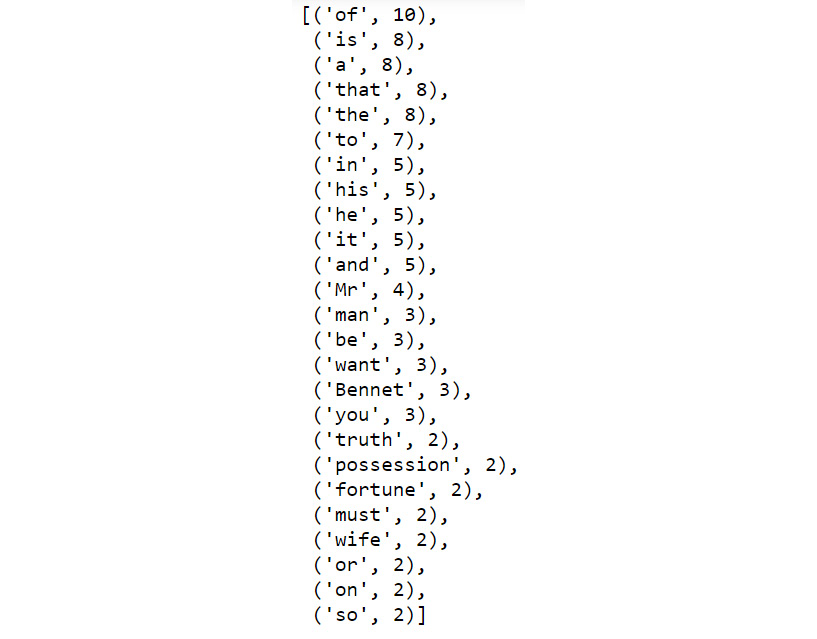Figure 1.19: Top 25 words from the unique list

Note

The solution for this activity can be found via this link.

# Summary

In this chapter, we learned about data wrangling and looked at examples from various real-life data science situations where data wrangling is very useful. We moved on to learn about the different built-in data structures that Python has to offer. We got our hands dirty by exploring lists, sets, dictionaries, tuples, and strings. These are the fundamental building blocks of Python data structures, and we need them all the time when working and manipulating data in Python. We did several small hands-on exercises to learn more about them. We finished this chapter with carefully designed activities that let us combine a lot of different tricks from all the different data structures and let us observe the interplay between all of them. In the next chapter, we will learn about the data structures in Python and utilize them to solve problems.

• ##### Brian Lipp

Brian Lipp is a technology polygot who is always in search of interesting and innovative technology. His current languages of choice are Python, Go, and Scala.

Browse publications by this author